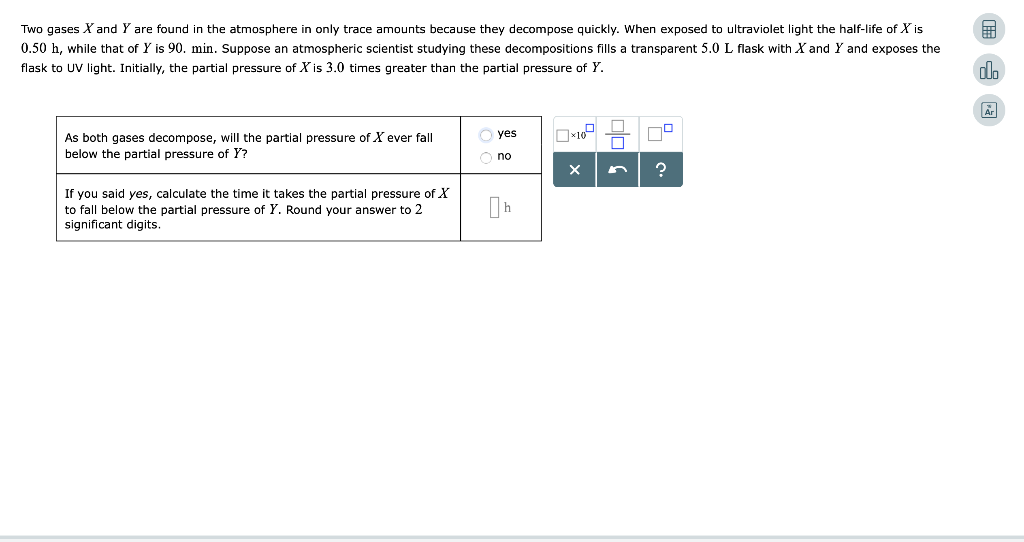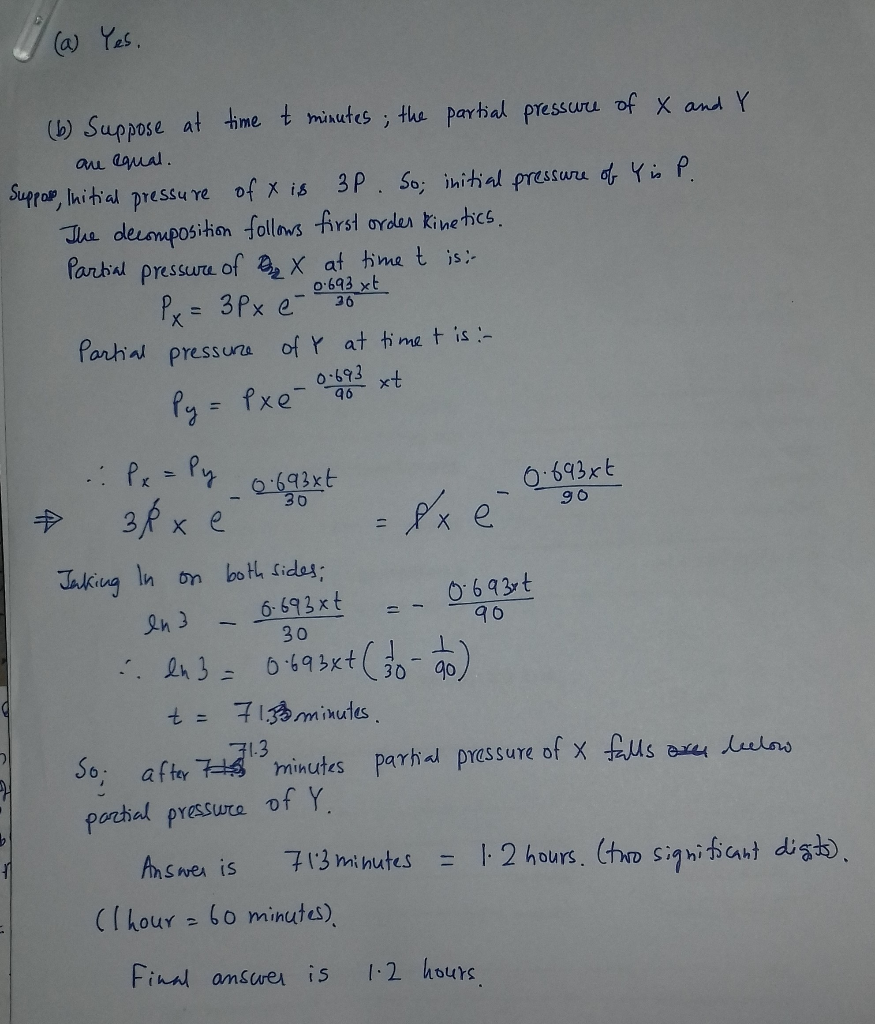# Two gases X and Y are found in the atmosphere only trace amounts because they decompose quickly. When exposed to ultrav...Two gases X and Y are found in the atmosphere only trace amounts because they decompose quickly. When exposed to ultraviolet light the half-life of Xis 0.50 h, while that of Y is 90. min. Suppose an atmospheric scientist studying these decompositions fills a transparent 5.0 L flask with Xand Y and exposes the flask to UV light. Initially, the partial pressure of X is 3.0 times greater than the partial pressure of Y. dlo yes As both gases decompose, will the partial pressure of X ever fall below the partial pressure of Y? no X If you said yes, calculate the time it takes the partial pressure of X to fall below the partial pressure of Y. Round your answer to 2 significant digits.##### Add Answer of: Two gases X and Y are found in the atmosphere only trace amounts because they decompose quickly. When exposed to ultrav...
More Homework Help Questions Additional questions in this topic.